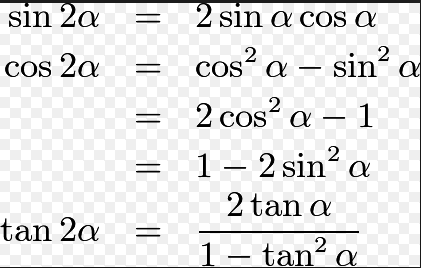# If in a triangle, ABC then the sides p, q and r Option 1) are in AP Option 2) are in GP Option 3) are in HP Option 4) satisfy p+q=r

As we discussed in concept

Double Angle Formula -- wherein

These are formulae for double angles.

=>

Option 1)

are in AP

This option is correct.

Option 2)

are in GP

This option is incorrect.

Option 3)

are in HP

This option is incorrect.

Option 4)

satisfy p+q=r

This option is incorrect.

### Preparation Products

##### Knockout BITSAT 2021

It is an exhaustive preparation module made exclusively for cracking BITSAT..

₹ 4999/- ₹ 2999/-